Courses

# Colligative Properties: Elevation In Boiling Point & Depression in Freezing Point Class 12 Notes | EduRev

## Class 12 : Colligative Properties: Elevation In Boiling Point & Depression in Freezing Point Class 12 Notes | EduRev

The document Colligative Properties: Elevation In Boiling Point & Depression in Freezing Point Class 12 Notes | EduRev is a part of the Class 12 Course Chemistry for JEE.
All you need of Class 12 at this link: Class 12

What is Boiling Point Elevation?

• Boiling point elevation refers to the increase in the boiling point of a solvent upon the addition of a solute.
• When a non-volatile solute is added to a solvent, the resulting solution has a higher boiling point than that of the pure solvent. For example, the boiling point of a solution of sodium chloride (salt) and water is greater than that of pure water.
• Boiling point elevation is a colligative property of matter, i.e. it is dependent on the solute-to-solvent ratio but not on the solute’s identity. This implies that the elevation in the boiling point of a solution depends on the amount of solute added to it.
• The greater the concentration of solute in the solution, the greater the boiling point elevation.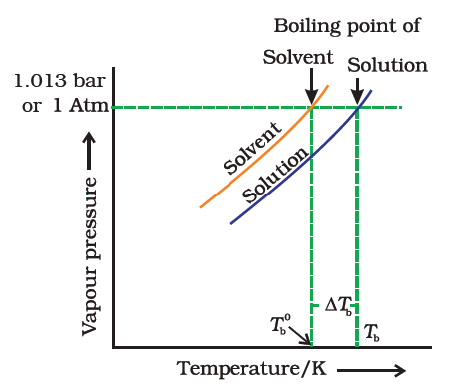Why Does Boiling Point Elevation Occur?

• Since the addition of a non-volatile solute causes a decrease in the vapour pressure of a solution, the boiling point of a solution is elevated as compared to the solvent i.e it must be heated to a higher temperature to make its vapour pressure equal to atmospheric pressure. Thus the solution boils at a higher temperature than the pure solvent.
• If Tb˚ is the boiling point of the solvent and Tb is the boiling point of the solution.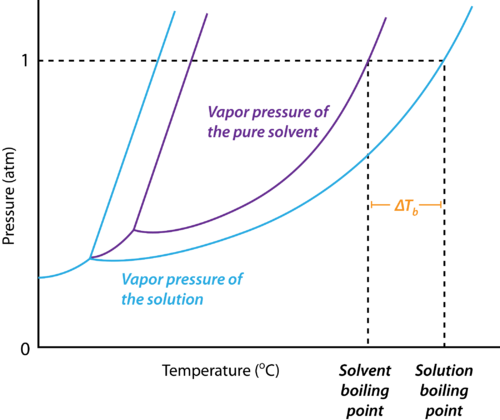Fig: Elevation in boiling point

We can see from the figure that the solution needs to be heated to a higher temperature to make it boil.

The difference between the boiling points of the solution and the solvent is known as the elevation in boiling point (ΔTb).

Elevation of boiling point, (ΔTb) = Boiling point of the solution - Boiling point of pure solvent
Elevation of boiling point, (ΔTb) = Tb -Tb˚
Experimentally, it is observed that elevation in boiling point is directly proportional to molal concentration of solute dissolved indefinite amount of the solvent.
ΔTb ∝ molality
ΔT= K×  molality .....(1)
ΔTb = Kb × m
Where Kb is proportionality constant and is known as the molal boiling point elevation constant or the ebullioscopic constant. It has the units of degree kg mol-1 or K kg mol-1.

Molality can be written as:

Molality (m) = Number of moles of solute/Mass of solvent in kg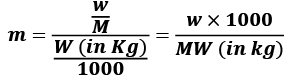w = mass of solute in grams
M = molecular wt of solute
W = mass of solvent in gram.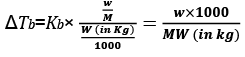Significance of Kb
Now if, m = 1 then,
ΔTb = Kb. Thus, the molal elevation constant Kb is defined as the elevation of the boiling point when the molality of the solution is one i.e. when 1 mole of the solute is dissolved in 1 kg of the solvent, the elevation of boiling point is equal of Kb.

Example 1. A 5 percent aqueous solution by mass of a non-volatile solute boils at 100.15°C. Calculate the molar mass of the solute. Kb = 0.52 K kg mol -1
Solution.
The normal boiling point of water is 100°C.
The elevation in boiling point is 0.15°C.
A 5 percent solution means that in a 100 g solution 5 g of the solute is present.
This implies that the solvent is 95 g.
If the molar mass of the solute is M, then the molality of the solute in the  solution is,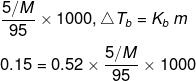M = 182.4 g/mol

Example 2. A solution of 12.5 g urea in 170 g of water gave a boiling point elevation of 0.63 K. Calculate the molar mass of urea, taking Kb = 0.52 K/m.
Solution.
Given: Mass of urea, w2 = 12.5 g
Mass of water, w1 = 170 g = 0.17 kg
Evaluation of boiling point, ΔTb = 0.63 K
Molar mass of urea, M = ?
Kb = 0.52 K m-1
Molar mass of the solute (urea) is given by: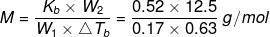= 60.7 g mol-1

Example 3. Calculate the molecular mass of a substance 1.0 g of which on being dissolved in 100 g of solvent gave an elevation of 0.307 K in the boiling point. (Molal elevation constant (Kb) = 1.84 K/m).
Solution.
We know that, ΔTb = , where n2 is the no. of moles of solute; w1 is the mass of the solvent in kg.
n2 = Mass of solute/Molar mass of the solute = 1.0 g/M and w1 = 100 g = 0.1 kg
Substituting these values, we get,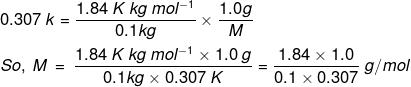= 59.9 g/mol

TRY YOURSELF!

Try yourself:The boiling point of chloroform is 61°C. After the addition of 5.0 g of a non-volatile solute to 20 g chloroform boils at 64.63°C. If kb = 3.63 K kg mol-1, what is the molecular weight of the solute?

What is Freezing Point Depression?

The freezing point of a substance is the temperature at which it's liquid and the solid phases have the same vapour pressure.

It is a colligative property of solutions that is generally proportional to the molality of the added solute. The depression in the freezing point of a solution can be described by the following formula: ΔT= iKf × m

Where ΔTf is the freezing point depression,
i is the Van’t Hoff factor,
Kf is the cryoscopic constant, and
m is the molality.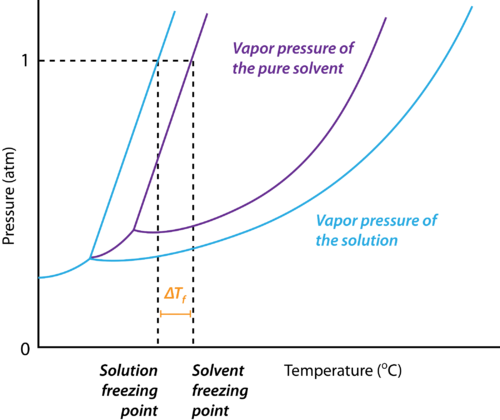Why does the Freezing Point Depression Occur?

The reason for the depression of the freezing point of a solvent upon the addition of a solute is explained below:

1. At the freezing point of a solvent, there exists an equilibrium between the liquid state and the solid-state of the solvent.
2. This implies that the vapour pressures of both the liquid and the solid phase are equal.
3. Upon the addition of a solute that is non-volatile, the vapour pressure of the solution is found to be lower than the vapour pressure of the pure solvent.
4. This causes the solid and the solution to reach equilibrium at lower temperatures.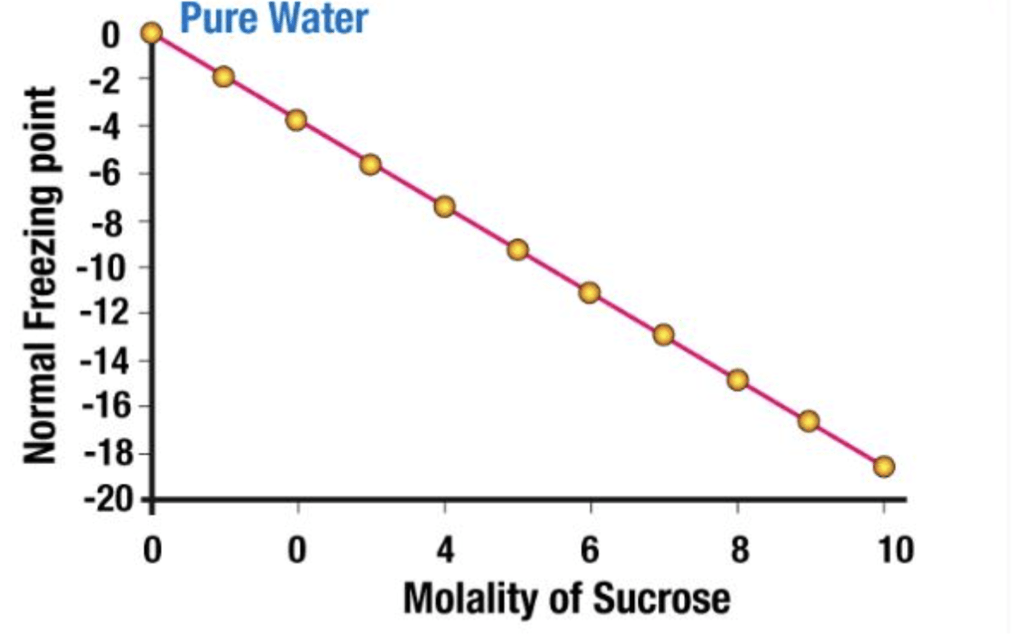If the difference between the freezing point of the solvent and the solution is represented as ΔTf,
Depression of Freezing point, (ΔTf) = Freezing point of the solution - Freezing point of pure solvent
Depression of Freezing point, (ΔTf) = Tf -Tf˚
Experimentally, it is observed that Depression in the Freezing point is directly proportional to the molal concentration of solute dissolved indefinite amount of the solvent.
ΔTf ∝ molality
ΔTf = Kf × molality   .....(1)
ΔT= Kf × m
Where Kf is proportionality constant and is known as the molal Depression point constant or the Cryoscopic constant. It has the units of degree kg mol-1 or K kg mol-1.

Molality can be written as

Molality (m) = Number of moles of Solute/Mass of Solvent in Kg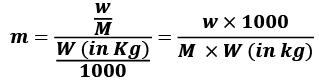w = mass of solute in grams
M = molecular wt of solute
W = mass of solvent in gram.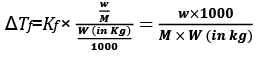Significance of Kf:
ΔT= Kfm. So, if m = 1 i.e. one mole of solute is dissolved in one kg of the solvent, then, ΔTf = Kf.
Thus,

The molal freezing point depression constant is equal to the depression in the freezing point produced when one mole of solute is dissolved in 1 kg of the solvent.

The values of molal elevation constant (Kb) for some common solvents are given in Table.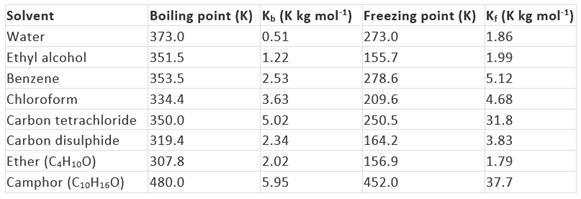Kb and Kf can be obtained from the following relationships: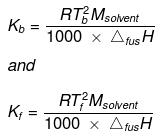Where, Tb = boiling point of the solvent,
T= freezing point of the solvent,
Msolvent = molar mass of the solvent,
ΔvapH = enthalpy of vaporization and
ΔfusH = enthalpy of fusion.

Key concept: Camphor is commonly used in determining the molecular mass of a solute because of its very high cryoscopic constant (Kf).

Example 1. An aqueous solution of a non-volatile solute boils at 100.17°C. At what temperature would it freeze? (Kb = 0.52 K kg mol - 1 and Kf = 1.88 K kg mol - 1).
Solution.
ΔTb = 0.17°C
m = ΔTb/K= 0.17/0.52
= 0.327 molal,
ΔTf = Kf × molality
ΔTf = 1.86 × 0.327 = 0.608
Freezing point of the solution is - 0.608°C.

Example 2. 1.4 g of (acetone dissolved) in 100 g of benzene gave a solution which freezes at 277.12 K. Pure benzene freezes at 278.4 . 2.8 g of a solid (A) dissolved in 100 g of benzene gave a solution which froze at 277.76 K. Calculate the molecular weight of (A).
Solution.

For acetone solute + Benzene solvent mixture:
ΔT = K'f × 1000 × w/M × W
(278.40 − 277.12) = 1000 × Kf × 1.4/100 × 58
or 1.28 = 1000 × Kf × 1.4/100 × 58 ...(i)
For solute (A) + Benzene mixture (Let M be the molecular Weight of A)
(278.40 − 277.76) = 1000 × Kf × 2.8/100 × M ...(ii)
By Eqs. (i) and (ii)
M = 232g

Example 3. Ethylene glycol (HOH2C - CH2OH) is used as an antifreeze for water to be used in car radiators in cold places. How much ethylene glycol should be added to 1 kg of water to prevent it from freezing at -10°C? Molal depression constant of water is 1.86 K kg mol-1
Solution.
Mass of water (solvent), w1 = 1 kg
ΔTf = 10°C Kf = 1.86 K kg mol-1
Mass of ethylene glycol required, w2 = ?
Molar mass of ethylene glycol = (12 + 3 +16) × 2 g/mol = 62 g/mol
We know, ΔTf = Kf(w2/62)/w1 or 10 = 1.86 × w2/62 x 1
This gives, w2 = 10 × 62 × 1/1.86 = 333.3 g

Example 4. Normal freezing point of a solvent is 150°C. A 0.5 molal solution of urea in the above solvent causes a freezing point depression of two degrees. Calculate the molal depression constant
Solution.
We know, ΔTf = Kfm
So, Kf = ΔTf/m = 2 deg/0.5 mol Kg-1 = 4 deg. kg mol-1

Example 5. A solution of urea in water freezes at 0.400°C. What will be the boiling point of the same solution if the depression and elevation constants for water are 1.86 deg kg mol-1 and 0.512 deg kg mol-1 respectively? Solution: We have the relationships:
Solution.
ΔTb = Kbn2/w1 ...(i)
And ΔTf = Kfn2/w1 ...(ii)
Where n2 is the number of moles of the solute, and w1 is the mass of solvent in kg.
Dividing equation (i) by equation (ii),
ΔTb/ΔTf = Kb/Kf
or,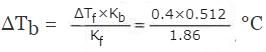= 0.11°C
Then, Boiling point of the urea solution = 100°C  + 0.11°C = 100.11°C.

TRY YOURSELF!

Try yourself:Pure CS2 melts at -112°C,  228 grams of propylene glycol crystals is mixed with 500 grams of CS2. If kf of CS2 = -3.83 K kg mol-1 what is the depression in the freezing point?

Offer running on EduRev: Apply code STAYHOME200 to get INR 200 off on our premium plan EduRev Infinity!

## Chemistry for JEE

196 videos|450 docs|369 tests

,

,

,

,

,

,

,

,

,

,

,

,

,

,

,

,

,

,

,

,

,

;